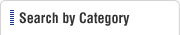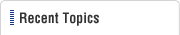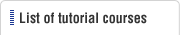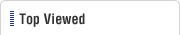HOME  > Press Dies Tutorial  > #127 Press Forming Force (4) Drawing Force
Press Dies Tutorial

#127 Press Forming Force (4) Drawing Force

Category : Drawing and Forming Work
June 8, 2012

The forming force (P) necessary for a cylindrical drawing is the force with which the punch pushes a circular blank into the die. The major factor related to the forming force is the resistance of the blank material to deformation. Apart from this, there is also the effect of the friction between the blank material and the die, the blank holding force (see course No. 10 for details of the blank holding force), etc. The sum of all these is the necessary drawing force.

The forming force of cylindrical drawing is determined very often using the following equation. Refer to Fig. 1.

 P = K * π * d * t * Ts (kgf) P: Drawing force (kgf) K: Coefficient π: Circumference ratio (3.14) d: Drawing diameter (mm) t: Plate thickness (mm) Ts: Tensile strength (kgf)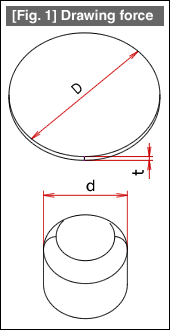The factors having large influences on the drawing force are the tensile strength of the material, the drawing ratio, relative plate thickness (defined as material plate thickness / blank diameter * 100 (%)).
When the drawing ratio is fixed, the coefficient (K) becomes smaller as the relative plate thickness becomes larger, and on the contrary, becomes larger as the relative plate thickness becomes smaller. Its limiting value is 1.0.
In the above equation, the material cracks when K exceeds 1.
If the relative plate thickness is fixed, it changes depending on the drawing ratio.
The coefficient is given for reference in the example of a steel plate (SPC).
The following is the value of K when the relative plate thickness is 1.2%.

###### (1) Initial drawing

Drawing ratio (m) = 0.50 → K = 1.0
0.55 → K = 0.80
0.60 → K = 0.68
0.70 → K = 0.42

###### (2) Redrawing

Drawing ratio (m) = 0.75 → K = 0.90
0.80 → K = 0.62
0.82 → K = 0.52
0.85 → K = 0.42
0.90 → K = 0.20

When the drawing ratio is the same in both initial drawing and redrawing, the K value will be larger in redrawing. This is due to the effect of work hardening of the material.
The drawing force obtained by calculation appears considerably above the bottom dead center of the press machine. The press used is selected considering the torque capacity of the press machine.
The press machine is not selected based on the relationship between the drawing force and the nominal capacity of the press machine.